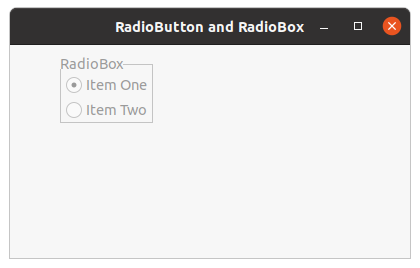# wxPython – Disable Radio Box present in frame

• Last Updated : 03 Jul, 2020

In this article we are going to learn that how can we disable a Radio Box present in the frame. In order to do that we will use Disable() method which makes the whole Radio Box disabled and unclickable. Disable() method returns True if the window has been disabled, False if it had been already disabled before the call to this function.

Disable() function takes no arguments.

Parameters No parameters required to Disable Radio Box

Returns: Returns True if the window has been disabled, False if it had been already disabled before the call to this function.

Return Type: bool

Code Example:

 `import` `wx`` ` ` ` `class` `FrameUI(wx.Frame):`` ` `    ``def` `__init__(``self``, parent, title):``        ``super``(FrameUI, ``self``).__init__(parent, title ``=` `title, size ``=``(``300``, ``200``))`` ` `        ``# function for in-frame components``        ``self``.InitUI()`` ` `    ``def` `InitUI(``self``):``        ``# parent panel for radio box``        ``pnl ``=` `wx.Panel(``self``)`` ` `        ``# list of choices``        ``hlist ``=` `[``'Item One'``, ``'Item Two'``]``        ``vlist ``=``[``'Item One'``, ``'Item Two'``]`` ` `        ``# create radio box with items in horizontal orientation``        ``self``.rbox ``=` `wx.RadioBox(pnl, label ``=``'RadioBox'``, pos ``=``(``50``, ``10``), choices ``=` `hlist,``                              ``majorDimension ``=` `0``, style ``=` `wx.RA_SPECIFY_ROWS)`` ` `        ``# create radio box with items in vertical orientation``        ``self``.rbox.Disable()`` ` `        ``# set frame in centre``        ``self``.Centre()``        ``# set size of frame``        ``self``.SetSize((``400``, ``250``))``        ``# show output frame``        ``self``.Show(``True``)`` ` ` ` ` ` `# wx App instance``ex ``=` `wx.App()``# Example instance``FrameUI(``None``, ``'RadioButton and RadioBox'``)``ex.MainLoop()`

Output Window:My Personal Notes arrow_drop_up